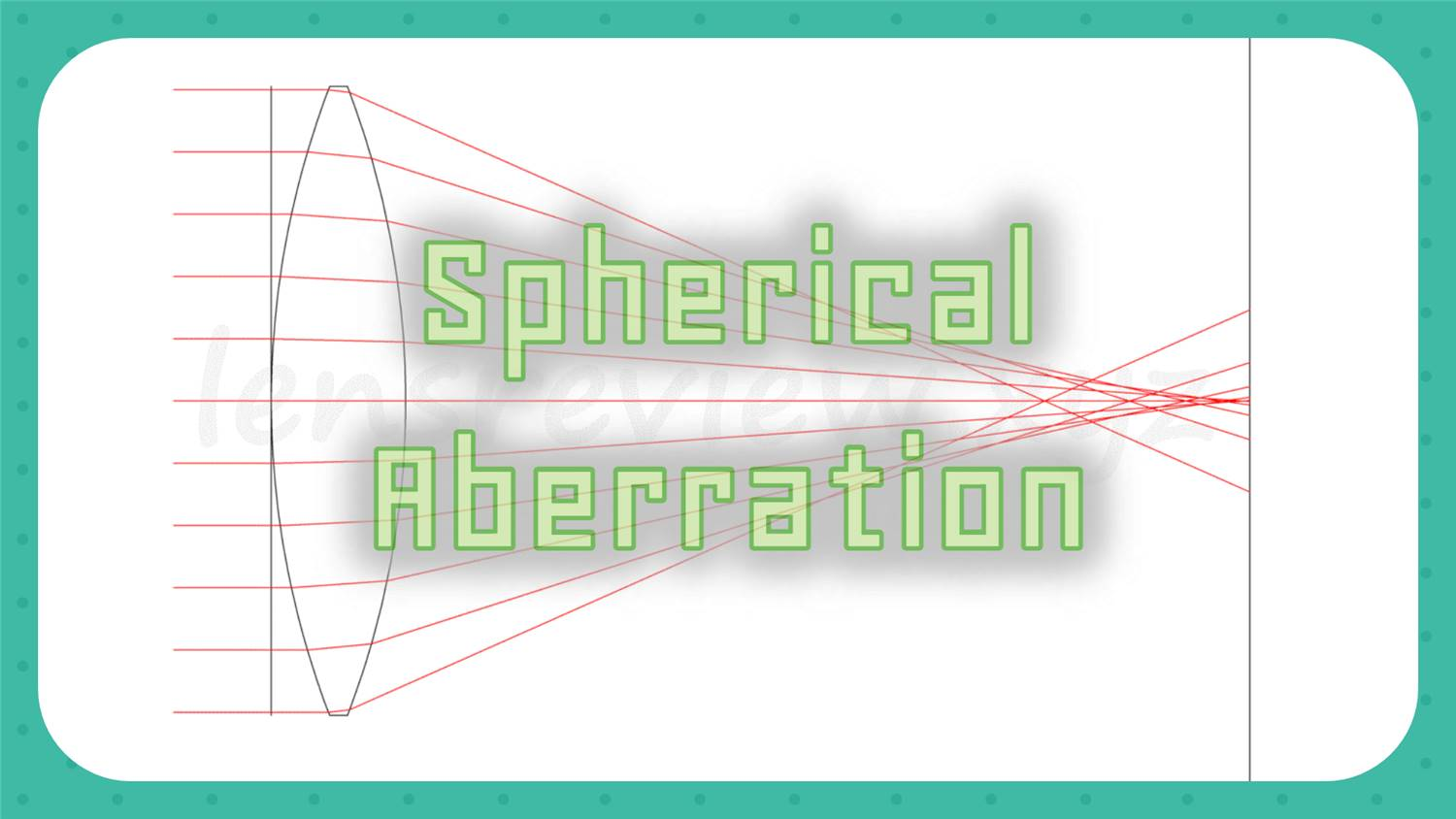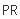KNOWLEDGE

# Spherical Aberration : A Guide for Photographers

2023-01-04

This series of articles conceptually explains aberration, which is a performance index of a lens.

Among aberrations, this section introduces "spherical aberration" in a simple and easy-to-understand manner using figures and simulations.

Introduction to Lens Design

## Aberrations and Spherical Aberrations

Aberration is a term used to describe the performance of a lens. It is an indicator of the deviation from an ideal imaging relationship, or in other words, the degree of light convergence.

The camera lens is designed to accurately focus the light emitted from the "subject" onto the imaging surface (film / COMS).

However, it is difficult to focus light accurately, and the small amount of error that inevitably deviates is called "aberration".

These aberrations are classified and named according to their characteristics.

If you are a little familiar with lenses, you have heard of spherical aberration.

Because "spherical aberration" is the most basic aberration.

First of all, when you hear "spherical aberration", you may imagine a difficult mathematical expression.

In this section, I would like to explain "spherical aberration" so that it can be understood as a concept as simple as possible.

## Let's imagine

Imagine that there is only one star in the pitch-dark sky, and you want to take it to the center of the picture.

This star is far away, many light-years away, so if you take a picture of it on the earth, you should be able to feel that it is an ideal picture to be taken as an "extremely small point of light" (ignoring the fluctuation of the air).

Unfortunately, depending on the lens performance, it may not be possible to focus on an ideal small point, resulting in a slightly blurred point.

The deviation from this ideal is the amount called "aberration", and the amount of blur in the center of the photo is the index value called "spherical aberration".

## Because the lens is round

The lens that collects the light is basically a sphere. (The lens is round.)

As a result, the light is collected more symmetrically (uniformly) in the center of the lens, and the light concentration in the center of the lens is the best in the entire screen.

Therefore, if you look at the performance of the center, you can immediately tell if it is a high-performance lens.

In other words, if the performance of the center is bad, you can't expect high performance.

For this reason, spherical aberration, which represents the performance of the center, is the basis of all aberrations and is considered to be the most important.

I will explain how spherical aberration looks in the optical path diagram posted in the lens analysis in this blog using a simulation.

The above example of "a star far away in the center of the screen" is shown as an optical path diagram below.

The image above shows a 50 mm F1.4 lens consisting of only one spherical lens.

Imagine using a cheap magnifying glass, such as one sold at a variety store, as a lens for your camera.

First, let's observe the light passing through the lens. (red line)

When light (red line) emitted from an ultra-distant light source, such as a star, hits the lens on the earth, it is positioned so that it is incident almost perpendicularly to the lens.

Light that passes through the lens travels toward the imaging plane (equivalent to film or CMOS) on the right side of the figure, and crosses in front of the image sensor.

It's very meaningful.

In addition, the star as the subject is far in the left direction of the screen about several light years, and it is difficult to draw, so it is omitted.

Collecting light from an extremely long distance is expressed as "collecting infinite light (or parallel light)".

In general, when expressing the aberration value of the lens of a camera, it is customary to express the aberration value in such a state of shooting distance that a distant star is photographed.

Let's take a closer look at the optical path diagram.

The usual way of drawing an optical path diagram is to draw only the light that passes through the outer diameter of the lens, so at a glance it appears as if the light is concentrated at a single point.

But in fact, the light passes through many magnifying lenses, as if it were an inexhaustible shower of fine lines.

So let's increase the number of lights we draw.

In the above illustration, the number of lights is increased. Normally, there is an infinite number of lights, but since I cannot write all of them, I have limited the number of lights to a level that is easy to see.

Now, if you look at the image pickup device (film surface) that collects light, it is not a perfect point.

It can be seen that the light passing through the center of the lens and the light passing through the outer periphery converge at different positions.

In other words, the aperture (Fno) of the lens is also related, but a single spherical lens cannot focus light on an ideal point due to the influence of "spherical aberration."

## Represented by an aberration diagram

Finally, I'm going to describe this single lens in the form of the spherical aberration diagram in our lens analysis article.

Now, the usual notation is as shown below.

### Longitudinal Aberration

Graphs of spherical aberration, field curvature, and distortion

The lens analysis article shows a graph of spherical aberration as one of the "longitudinal aberrations".

We place the spherical aberration diagram as the first graph in the article because it is the most important graph.

The explanatory diagram of the arrangement of longitudinal aberration and spherical aberration is here.

The horizontal axis scale of the aberration chart in the lens analysis article is ± 0.5 mm, but the aberration amount of this single lens is too large and the horizontal axis scale is ± 10 mm. Please note that it is 20 times the normal value.

Now, what does the vertical / horizontal axis mean in this spherical aberration diagram? The article continues.

## Illustration: Spherical Aberration

Let me illustrate the optical path diagram and spherical aberration a little more simply.

The relationship between the optical path diagram and spherical aberration can be expressed simply as shown below.

Spherical aberration To explain the structure of the diagram, the vertical and horizontal axes that make up the graph

• Horizontal axis: focusing position (out of focus) for each light
• Vertical axis: the incident position (height) of the light beam

Can you see the relationship between the vertical axis and the horizontal axis of the spherical aberration graph?

You can read many things from this graph.

For example, a lens with a large spherical aberration appears to be out of focus, but there are numerous focal points, so it cannot be expressed by a single point.

A lens with a bright Fno has a high ray height, i.e., a long vertical axis of spherical aberrations, and the brighter the Fno, the greater the spherical aberrations. I can foresee such a thing.

## Compensate for the Spherical Aberration

Up until a while ago, the explanation was made with one lens, but in reality, such a bright optical system of Fno consists of many lenses.

Let's look at the characteristics of the lens corrected for spherical aberration.

Earlier, I introduced the aberration of 50mm F1.4 lens composed of only one lens.

The figure below shows the spherical aberration of a double Gaussian type 7-piece lens on the same spherical aberration graph scale.

### Optical path diagram

The configuration shown in the optical path diagram is shown above.

This lens is the NIKON NIKKOR 50mm f1.4D which was analyzed in the past.

### Longitudinal Aberration

Spherical aberration, field curvature, and distortion

The graph is made in 20 times the normal size according to the horizontal axis scale of the first single-component lens.

Looking at the spherical aberration diagram, there is a question as to whether it was necessary to display it as an approximate straight line, but although this lens is in the lowest price class, it utilizes the characteristics of the double Gaussian type lens, which is the crystal of human wisdom, and is able to correct aberrations with a very small number of lenses, so you can understand how amazing it is.

Check out the original article if you want to see it in its usual graph scale.

NIKON NIKKOR 50 mm f1.4D

## Conclusion

To put it simply, spherical aberration is the blurring of an image in the center of the screen.

I'm sure that your understanding of spherical aberration has made our blog 20 times more enjoyable.